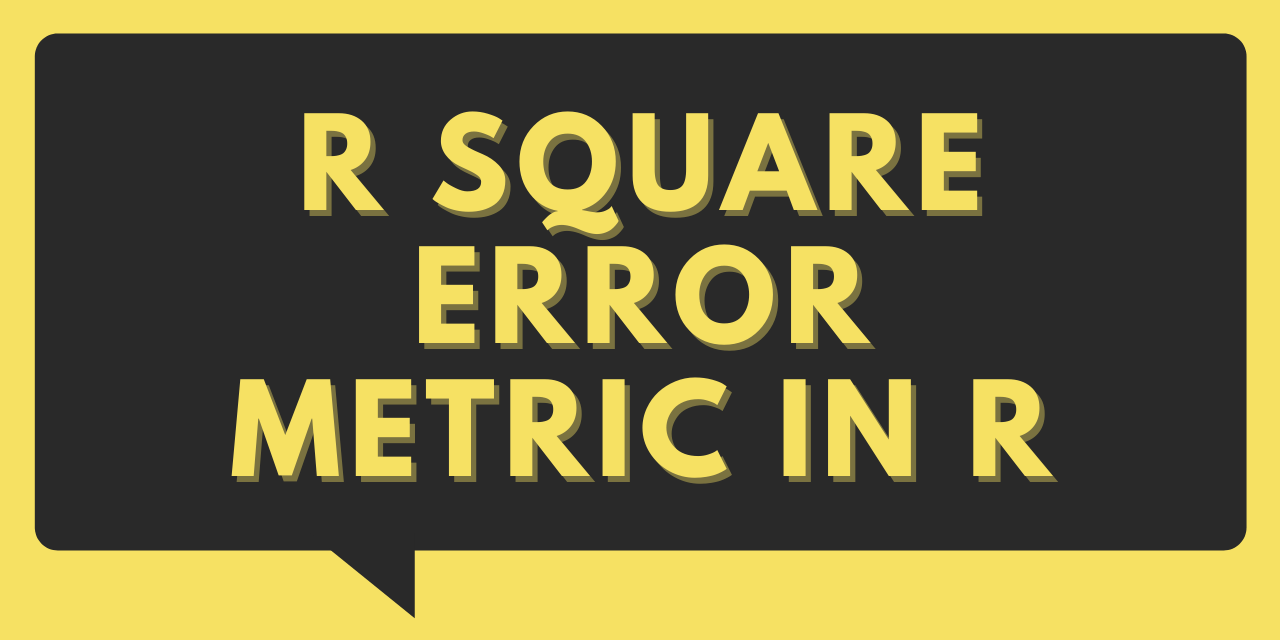# R Squared in R - How to Calculate R2 in R?

Published on August 3, 2022By Safa MulaniWhile we believe that this content benefits our community, we have not yet thoroughly reviewed it. If you have any suggestions for improvements, please let us know by clicking the “report an issue“ button at the bottom of the tutorial.

Hello, readers! In this article, we would be walking through an important concept in Machine Learning - R squared (R2) in R programming.

So, let us begin!!

## Importance of R squared error metric

Let us first understand the importance of error metrics in the domain of Data Science and Machine Learning!!

Error metrics enable us to evaluate the performance of a machine learning model on a particular dataset.

There are various error metric models depending upon the class of algorithm.

We have the Confusion Matrix to deal with and evaluate Classification algorithms. While R square is an important error metric to evaluate the predictions made by a regression algorithm.

`R squared (R2)` is a regression error metric that justifies the performance of the model. It represents the value of how much the independent variables are able to describe the value for the response/target variable.

Thus, an R-squared model describes how well the target variable is explained by the combination of the independent variables as a single unit.

The R squared value ranges between 0 to 1 and is represented by the below formula:

R2= 1- SSres / SStot

Here,

• SSres: The sum of squares of the residual errors.
• SStot: It represents the total sum of the errors.

Always remember, Higher the R square value, better is the predicted model!

## I. R-Squared in R with Linear Regression

In this example, we have implemented the concept of R square error metric on the Linear Regression model.

2. The next step is to segregate the data into training and test datasets. This is achieved using `createDataPartition()` method.
3. Before modeling, we have specified the custom functions for our error metrics as seen in the below example.
4. The last step is to apply the linear regression model using `lm()` function and then we have called the user-defined R square function to evaluate the performance of the model

Example:

``````#Removed all the existing objects
rm(list = ls())
#Setting the working directory
setwd("D:/Ediwsor_Project - Bike_Rental_Count/")
getwd()

### SAMPLING OF DATA -- Splitting of Data columns into Training and Test dataset ###
categorical_col_updated = c('season','yr','mnth','weathersit','holiday')
library(dummies)
bike = bike_data
bike = dummy.data.frame(bike,categorical_col_updated)
dim(bike)

#Separating the depenedent and independent data variables into two dataframes.
library(caret)
set.seed(101)
split_val = createDataPartition(bike\$cnt, p = 0.80, list = FALSE)
train_data = bike[split_val,]
test_data = bike[-split_val,]

### MODELLING OF DATA USING MACHINE LEARNING ALGORITHMS ###
#Defining error metrics to check the error rate and accuracy of the Regression ML algorithms

#1. MEAN ABSOLUTE PERCENTAGE ERROR (MAPE)
MAPE = function(y_actual,y_predict){
mean(abs((y_actual-y_predict)/y_actual))*100
}

#2. R SQUARED error metric -- Coefficient of Determination
RSQUARE = function(y_actual,y_predict){
cor(y_actual,y_predict)^2
}

##MODEL 1: LINEAR REGRESSION
linear_model = lm(cnt~., train_data) #Building the Linear Regression Model on our dataset
summary(linear_model)
linear_predict=predict(linear_model,test_data[-27]) #Predictions on Testing data

LR_MAPE = MAPE(test_data[,27],linear_predict) # Using MAPE error metrics to check for the error rate and accuracy level
LR_R = RSQUARE(test_data[,27],linear_predict) # Using R-SQUARE error metrics to check for the error rate and accuracy level
Accuracy_Linear = 100 - LR_MAPE

print("MAPE: ")
print(LR_MAPE)
print("R-Square: ")
print(LR_R)
print('Accuracy of Linear Regression: ')
print(Accuracy_Linear)

``````

Output:

As seen below, the R square value is 0.82 i.e. the model has worked well for our data.

``````> print("MAPE: ")
 "MAPE: "
> print(LR_MAPE)
 17.61674
> print("R-Square: ")
 "R-Square: "
> print(LR_R)
 0.8278258
> print('Accuracy of Linear Regression: ')
 "Accuracy of Linear Regression: "
> print(Accuracy_Linear)
 82.38326
``````

## II. R square value using summary() function

We can even make use of the `summary() function` in R to extract the R square value after modelling.

In the below example, we have applied the linear regression model on our data frame and then used `summary()\$r.squared` to get the r square value.

Example:

``````rm(list = ls())

A <- c(1,2,3,4,2,3,4,1)
B <- c(1,2,3,4,2,3,4,1)
a <- c(10,20,30,40,50,60,70,80)
b <- c(100,200,300,400,500,600,700,800)
data <- data.frame(A,B,a,b)

print("Original data frame:\n")
print(data)

ml = lm(A~a, data = data)

# Extracting R-squared parameter from summary
summary(ml)\$r.squared

``````

Output:

`````` "Original data frame:\n"
A B  a   b
1 1 1 10 100
2 2 2 20 200
3 3 3 30 300
4 4 4 40 400
5 2 2 50 500
6 3 3 60 600
7 4 4 70 700
8 1 1 80 800

 0.03809524
``````

## Conclusion

By this, we have come to the end of this topic. Feel free to comment below, in case you come across any question.

Till then, Happy Learning!! :)

Thanks for learning with the DigitalOcean Community. Check out our offerings for compute, storage, networking, and managed databases.Safa Mulani

author

#### Still looking for an answer?

Ask a questionSearch for more help

Click below to sign up and get \$200 of credit to try our products over 60 days!

### Popular Topics##### Hollie's Hub for Good

Working on improving health and education, reducing inequality, and spurring economic growth? We’d like to help.##### Become a contributor

You get paid; we donate to tech nonprofits.

##### Welcome to the developer cloud

DigitalOcean makes it simple to launch in the cloud and scale up as you grow – whether you’re running one virtual machine or ten thousand.© 2023 DigitalOcean, LLC.